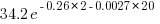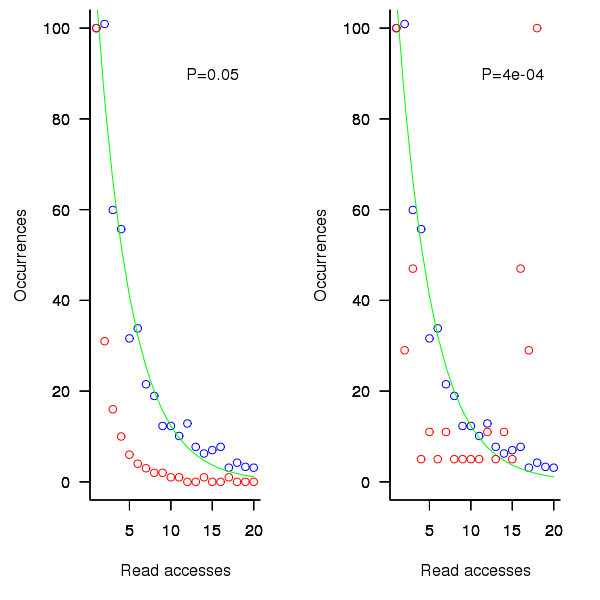[This article was first published on The Shape of Code » R, and kindly contributed to R-bloggers]. (You can report issue about the content on this page here)
Want to share your content on R-bloggers? click here if you have a blog, or here if you don't.

If, when writing code for a function, up to the current point in the codedistinct local variables have been accessed for readingtimes (), will the next read access be from a previously unread local variable and if not what is the likelihood of choosing each of the distinctvariables (global variables are ignored in this analysis)?

• With probabilityselect a new variable to access,
• otherwise select a variable that has previously been accessed in the function, with the probability of selecting a particular variable being proportional to(whereis the number of times the variable has previously been read from.

The longer answer is below as another draft section from my book Empirical software engineering with R. As always comments and pointers to more data welcome. R code and data here.

The discussion on preferential attachment is embedded in a discussion of model building.

# What kind of model to build?

The obvious answer to the question of what kind of model to build is, the cheapest one that produces the desired output.

Many of the model building techniques discussed in this book find patterns in the data and effectively return one or more equations that produce output similar to the data given some set of inputs; the equations are the model.

The advantage of this approach is that in many cases the implementation of the model building has been automated (I don’t say much about those that have not yet been automated), the user contribution is in choosing which kind of model to build. In some cases the R function requires that the user provide a general direction of attack (e.g., the form of function to use in fitting a nonlinear regression).

An alternative kind of model is one whose output is obtained by running an iterative algorithm, e.g., a time series created by calculating the next value in a sequence from one or more previous values.

In most cases a great deal of domain knowledge is required of the user building the model, while in a few cases an automated procedure for creating the iterative algorithm and its parameters is available, e.g., time series analysis.

There is never any guarantee that any created model will be sufficiently accurate to be useful for the problem at hand; this is a risk that occurs in all model building exercises.

The following discussion builds two models, one using an established automated model building technique (regression) and the other using an iterative algorithm built using domain knowledge coupled with experimentation.

The problem
Consider local variable usage within a function. If a function contains a total ofread accesses to locally defined variables, how many variables will be read from only once, how many twice and so on (this is a static count extracted from the source code, not a dynamic count obtained by executing the function)?

The data for the following analysis is from Jones (see figure 1821.5) and contains three columns, total count: the total number of read accesses to all variables defined within a function definition, object access: the number of read accesses from a distinct local variable, and occurrences: the number of distinct variables that have at least one read access within the function (i.e., unused variables are not counted); the occurrences counts have been summed over all functions.

In the following extract, within functions containing 24 totals accesses there were 783 occurrences of variables accessed once, 697 occurrences of variables accessed twice and so on.

 ```total access,object access,occurrences 24,1,783 24,2,697 24,3,474```

The data excludes everything about source code apart from read access information.

Fitting an equation to the data
Plotting the data shows an exponential-like decrease in occurrences as the number of accesses to a variable increases (i.e., most variables are accessed a small number of time); also there is an overall increase in the counts as the total numbers of accesses increases (see below).

The fit obtained by the `nls` function for a simple exponential equation is the following (all p-values less than; see rexample[local-use]):

whereis the number of read accesses to a given variable andis the total accesses to all local variables within the function. Because the data has been normalised the value returned is a percentage.

As an example, a function containing a total of 30 read accesses of local variables the expected percentage of variables accessed twice is:.

Modeling with an incremental algorithm
If, when writing code for a function, up to the current point in the codedistinct local variables have been accessed for readingtimes (), will the next read access be from a previously unread local variable and if not what is the likelihood of choosing each of the distinctvariables (global variables are ignored in this analysis)?

Each access in the code of a local variable could be thought of as a link to the information contained in that variable. One algorithm that has been found to do a reasonable job of modeling the number of links between web pages is Preferential attachment. Might this algorithm also be applicable to modeling read accesses to local variables?

The Preferential attachment algorithm is:

• With probabilityselect a new web page (in this case a new variable to access),
• with probabilityselect an existing web page (a variable that has previously been accessed in the function), select a variable with a probability proportional to the number of times it has previously been accessed (i.e., a variable that has four previous read accesses is twice as likely to be chosen as one that has had two previous accesses).

The following plot shows the results of running this algorithm 1,000 times each with 100 total accesses per function definition, for two values of(left plot 0.05, right plot 0.0004, red points) and smoothed data (blue points; smoothing involved summing the access counts for all measured functions having total accesses between 96 and 104), green line is a fitted exponential. Values have been normalised so that variables with one access have a count of 100, also access counts greater than 20 have a very low occurrences and are not plotted.Figure 1. Variables having a given number of read accesses, given 100 total accesses, calculated from running the preferential attachment algorithm with probability of accessing a new variable at 0.05 (left, in red) and 0.0004 (right, in red), the smoothed data (blue) and a fitted exponential (green).

The results show that decreasing the probability of accessing a new variable,, does not shift the distribution of occurrences in the desired way. Note: the well known analytic solution to the outcome of running the preferential attachment algorithm, i.e., a power law, applies in the situation where the number of accesses per function definition goes to infinity.

The Preferential attachment algorithm uses a fixed probability for deciding whether to access a new variable; other measurements imply that in practice this probability decreases as the number of distinct local variables increases. An obvious modification is to use a probability having a form something like

the number of distinct variables accessed so far). A little experimentation finds thatproduces results that more closely mimic the data.

Whileimproves the fit for infrequently accessed variables, the weighting system used to select a previously accessed variable still needs attention; perhaps it also has a dependency on. Some experimentation finds that changing the probability of selection fromto(whereis the number of read accesses to variableso far) produces behavior that matches the data to the same degree as the exponential model.Figure 2. Variables having a given number of read accesses, given 25, 50, 75 and 100 total accesses, calculated from running the weighted preferential attachment algorithm (red), the smoothed data (blue) and a fitted exponential (green).

The weighted preferential attachment algorithm is as follows:

• With probabilityselect a new variable to access,
• with probabilityselect a variable that has previously been accessed in the function, select an existing variable with probability proportional to(whereis the number of times the variable has previously been read from; e.g., if the total accesses up to this point in the code is 12, a variable that has had four previous read accesses istimes as likely to be chosen as one that has had two previous accesses).

So what?
Both of the models are wrong in that they do not account for the small number of very frequently accessed variables that regularly occur in the data. However, as the adage goes: All models are wrong but some are useful; usefulness being evaluated by the extent to which a model solves the problem at hand. Both models have their own advantages and disadvantages, including:

• the fitted equation is quick and simple to calculate, while the output from the algorithmic model has to be averaged over many runs (1,000 are used in the example code) and is much slower,
• the algorithm automatically generates a possible sequence of accesses, while the equation does not provide an obvious way for generating a sequence of accesses,
• multiple executions of the algorithm can be used to obtain an estimate of standard deviation, while the equation does not provide a method for estimating this quantity (it may be possible to build another regression model that provides this information),

If insight into variable usage is the aim, each model provides its own particular kind of insight:

• the equation provides an end result way of thinking about how the number of variables having a given number of accesses changes, but does not provide any insight into the decision process at the level of individual accesses,
• the algorithm provides a way of thinking about how choices are made for each access, but does not provide any insight into the behavior of the final counts.

Other application domains and languages
The data used to build these models was extracted from the C source code of what might be termed desktop applications. Will the same variable access behavior characteristics occur in source written for other application domain or in other languages?

Variables might be broadly grouped into those used to hold application values (e.g., length of something) and those used to hold housekeeping values (e.g., loop counters).

Application variables are likely to be language invariant but have some dependence on algorithm (e.g., stored in an array or linked list) or cultural coding habits (e.g., within the embedded community accessing local variables is often considered to be much less efficient than accessing global variables and there are measurably different usage patterns figure 288.1).

The need for housekeeping values will depend on the construct supported by a language. For instance, in C loops often involve three accesses to the loop control variable to initialise, increment and test it ` for (i=0; i < 10; i++)`; in languages that support usage of the form ` for (i in v_list)` only one access is required; in languages with vector operations many loops are implicit.

It is possible that application and language issues will change the absolute number of accesses but not effect their distribution. More measurements are needed.